# ISEE Middle Level Math : Distributive Property

## Example Questions

1 2 3 4 5 6 7 8 9 11 Next →

### Example Question #101 : Distributive Property

Solve using the distributive property: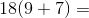Possible Answers: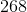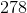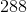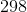Correct answer:Explanation:

To solve, multiply each number inside the parentheses by 18: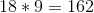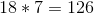Then solve: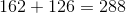Answer:### Example Question #102 : Distributive Property

Solve using the distributive property: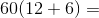Possible Answers: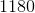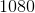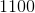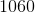Correct answer:Explanation:

To solve, multiply each number inside the parentheses by 60: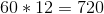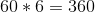Then, solve: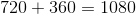Answer:### Example Question #103 : Distributive Property

Which of the following is equivalent to this expression?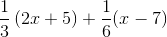Possible Answers: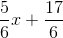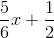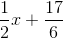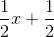Correct answer:Explanation: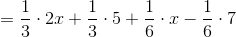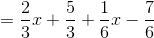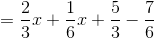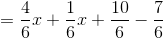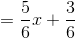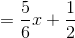### Example Question #104 : Distributive Property

Which of the following is equivalent to this expression?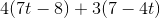Possible Answers: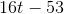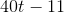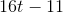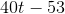Correct answer:Explanation:

Using the distributive property: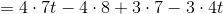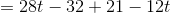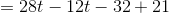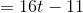### Example Question #105 : Distributive Property

Which of the following is equivalent to this expression?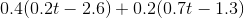Possible Answers: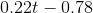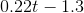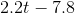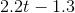Correct answer:Explanation:

To find an equivalent expression first distribute the terms outside of the parentheses to each term within the parentheses.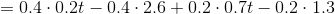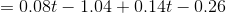After distributing the terms and performing the multiplication, combine the like terms.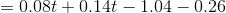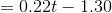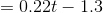### Example Question #106 : Distributive Property

Simplify the following: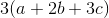Possible Answers: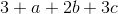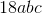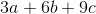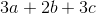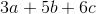Correct answer:Explanation:

To distribute, we will take the coefficient in front, and multiply each term by it.

So, we get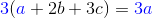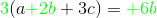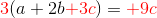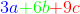### Example Question #107 : Distributive Property

Simplify the following terms: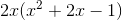Possible Answers: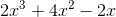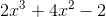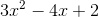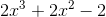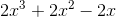Correct answer:Explanation:

Use the distributive property to simplify the expression.

Multiply the monomial with the terms of the trinomial.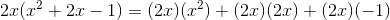Expand the terms.

The answer is:1 2 3 4 5 6 7 8 9 11 Next →

### All ISEE Middle Level Math Resources